## TyroCity

Physics XII Notes for Physics Notes

Posted on • Updated on

# Torque On Rectangular Coil In A Magnetic Field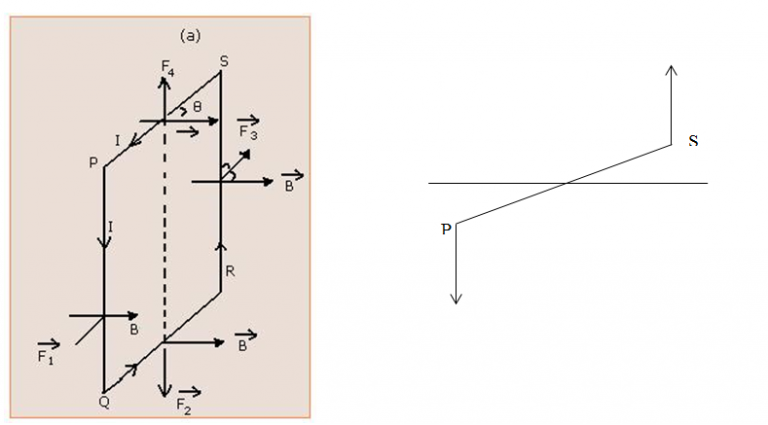As the current carrying conductor experiences a force when placed in a magnetic field, each side of a current carrying rectangular coil experiences a force in a magnetic field. In the present section we shall see in what way the rectangular loop carrying current is influenced by a magnetic field.

Consider a rectangular coil of length l and breadth b carrying a current I placed in a uniform magnetic field B. θ be the angle between plane of rectangular coil and magnetic field. The magnitude of experienced by each side of loop is given below.

- Force acting on side PQ (F1):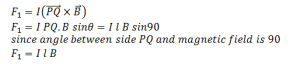The direction of force is perpendicular to the plane containing PQ and B. It is directed outward direction as shown in figure.

- Force acting on side QR (F2):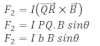The direction of force is perpendicular to the plane containing QR and B. It is directed downward direction as shown in figure.

- Force acting on side RS (F3):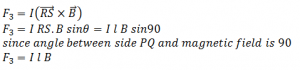The direction of force is perpendicular to the plane containing RS and B. It is directed inward direction as shown in figure.

- Force acting on side SP (F4):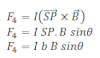The direction of force is perpendicular to the plane containing SP and B. It is directed upward direction as shown in figure.

As the force acting on the upper and lower sides are equal and opposite along the same line of action, they cancel each other. As the force acting on the sides QR and SP are equal and opposite along different lines of action they constitute a couple. Hence the rectangular coil experiences a torque.

Therefore the magnitude of torque acting on the coil is: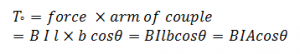If the rectangular coil having N number of turns then torque is given by: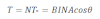Thus the torque acting on a coil in a magnetic field depends on the number of turns, area of current loop, strength of current and magnetic field.